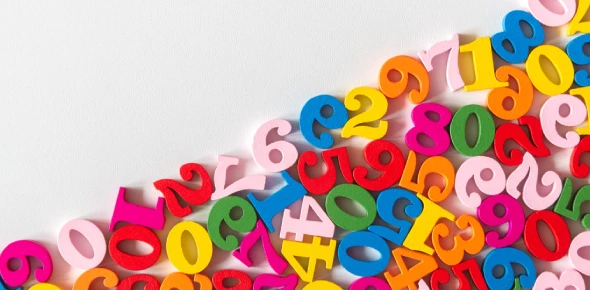# Simple Mathematics Questions Related To Number

Approved & Edited by ProProfs Editorial Team
At ProProfs Quizzes, our dedicated in-house team of experts takes pride in their work. With a sharp eye for detail, they meticulously review each quiz. This ensures that every quiz, taken by over 100 million users, meets our standards of accuracy, clarity, and engagement.
| Written by Janine
J
Janine
Community Contributor
Quizzes Created: 1 | Total Attempts: 71
Questions: 14 | Attempts: 71SettingsMathematics is a very fun subject when one gives it a chance. Do accurately understand the concept of place values, odd and even numbers? The test below is designed to test what you understand about these concepts and basic mathematic functions. Give it a try and check out your score.

• 1.

### What is the value of 8 in 8996?

• A.

8 thousand

• B.

8 hundred

• C.

8 ten thousand

• D.

8 million

A. 8 thousand
Explanation
The value of 8 in 8996 is 8 thousand because the number 8 is in the thousands place.

Rate this question:

• 2.

### What is the value of 8 in 6768?

• A.

8 hundred

• B.

8 tens

• C.

8 ones/units

• D.

8 hundred thousands

C. 8 ones/units
Explanation
In the number 6768, the value of 8 is in the ones/units place. This means that there are 8 individual units represented by the digit 8 in the number.

Rate this question:

• 3.

### 1, 3, 5, 7, 9 are

• A.

Even numbers

• B.

Odd numbers

• C.

Ordinal number

• D.

Counting numbers

B. Odd numbers
Explanation
The given sequence 1, 3, 5, 7, 9 consists of numbers that are not divisible by 2, which means they are not even numbers. Instead, they are all odd numbers as they are not multiples of 2 and can be obtained by adding 2 successively to the previous number in the sequence.

Rate this question:

• 4.

### 13, 7, 21, 19 are

• A.

Odd numbers

• B.

Counting numbers

• C.

Even numbers

• D.

Ordinal numbers

A. Odd numbers
Explanation
The given sequence 13, 7, 21, 19 consists of numbers that are not divisible by 2, hence they are odd numbers.

Rate this question:

• 5.

### 2nd, 5th, 10th are

• A.

Ordinal numbers

• B.

Even numbers

• C.

Counting numbers

• D.

Odd numbers

A. Ordinal numbers
Explanation
The numbers mentioned (2nd, 5th, 10th) are indicating the position or order of something in a sequence. Ordinal numbers are used to represent the position of an object or an element in a series. In this case, the numbers are being used to indicate the position of something, hence they are ordinal numbers.

Rate this question:

• 6.

### 19 rounded off to the nearest ten is

• A.

10

• B.

18

• C.

20

• D.

21

C. 20
Explanation
When rounding off a number to the nearest ten, we look at the digit in the ones place. If the digit is 5 or greater, we round up. If the digit is less than 5, we round down. In this case, the digit in the ones place is 9, which is greater than 5. Therefore, we round up to the nearest ten, which is 20.

Rate this question:

• 7.

### 241 rounded off to the nearest hundred is

• A.

200

• B.

250

• C.

240

• D.

300

A. 200
Explanation
When rounding off a number to the nearest hundred, we look at the tens digit. In this case, the tens digit is 4. Since it is less than 5, we round down to the nearest hundred. Therefore, 241 rounded off to the nearest hundred is 200.

Rate this question:

• 8.

### 6819 rounded off to the nearest thousand is

• A.

6800

• B.

6900

• C.

7000

• D.

6000

C. 7000
Explanation
When rounding off a number to the nearest thousand, we look at the digit in the hundreds place. If it is 5 or greater, we round up, and if it is less than 5, we round down. In this case, the digit in the hundreds place is 8, which is greater than 5. Therefore, we round up to the nearest thousand, which is 7000.

Rate this question:

• 9.

### 4 2 6 -  38

• A.

388

• B.

488

• C.

256

• D.

885

A. 388
Explanation
The given sequence is a combination of the numbers 4, 2, and 6. By rearranging these numbers, we can form the number 388, which is the correct answer.

Rate this question:

• 10.

• A.

554

• B.

468

• C.

485

• D.

338

B. 468
• 11.

### 300-136

• A.

164

• B.

224

• C.

398

• D.

154

A. 164
Explanation
The given sequence of numbers follows a specific pattern. Each number in the sequence is obtained by subtracting 136 from the previous number. Starting with 300, subtracting 136 gives us 164. Therefore, 164 is the next number in the sequence.

Rate this question:

• 12.

### 15*8

• A.

130

• B.

220

• C.

120

• D.

320

C. 120
Explanation
The correct answer is 120 because when you multiply 15 by 8, you get the product of 120.

Rate this question:

• 13.

### 81*5

• A.

405

• B.

325

• C.

555

• D.

425Back to top